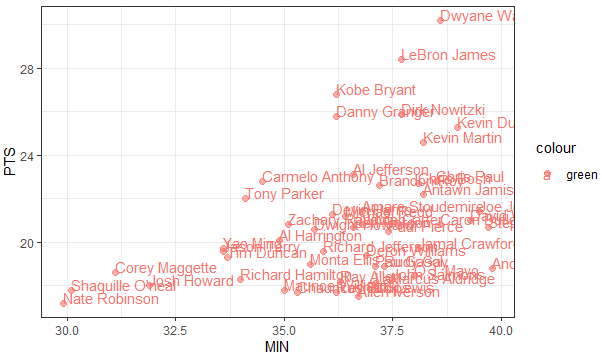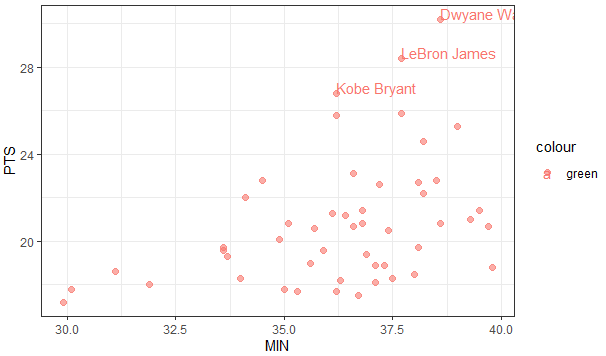5 views

The data I'm playing with comes from the internet source listed below

What I want to do, is create a 2D points graph comparing two metrics from this table, with each player representing a dot on the graph. I have the following code:

nbaplot <- ggplot(nba, aes(x= MIN, y= PTS, colour="green", label=Name)) +

geom_point()

This gives me the following:What I want is a label of the player's name right next to the dots. I thought the label function in ggplot's aesthetics would do this for me, but it didn't.

I also tried text() function and the textxy() function from the library(calibrate), neither of which appears to work with ggplot.

How can I add name labels to these points?

by

You can add the geom_text() function to add labels to the points as follows:

ggplot(nba, aes(x= MIN, y= PTS, colour="green", label=Name)) +

geom_point(size = 2,alpha = 0.6) +

theme_bw()+

geom_text(aes(label=Name),hjust=0, vjust=0)

Output:To only label points above a certain value:

ggplot(nba, aes(x= MIN, y= PTS, colour="green", label=Name)) +

geom_point(size = 2,alpha = 0.6) +

theme_bw()+

geom_text(aes(label=ifelse(PTS>26,as.character(Name),'')),hjust=0,vjust=0)

Output:+1 vote